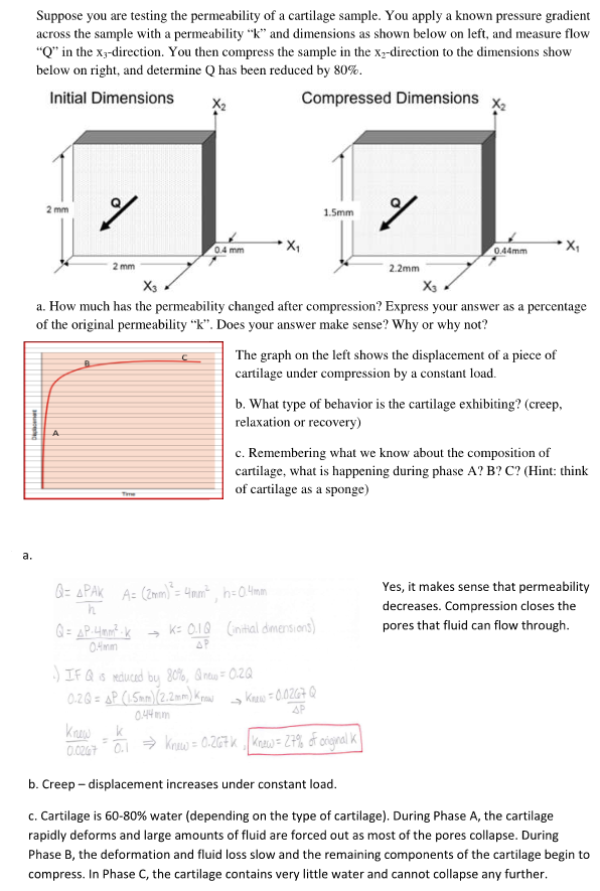Suppose you are testing the permeability of a cartilage sample. You apply a known pressure gradient across the sample with a permeability k and dimensions as shown below on left, and measure flow Q in the xy direction. You then compress the sample in the x2 direction to the dimensions shown below on right, and determine Q has been reduced by 80 percent. a) How much has the permeability changed after compression? Express your answer as a percentage of the original permeablity k. Does your answer make sense? Why or why not? The graph on the left shows the displacement of a piece of cartilage under compression by a constant load. b) What type of behavior is the cartilage exhibiting? c) Remembering what we know about the composition of cartilage, what is happening during phase A? B? C?Suppose you are testing the permeability of a cartilage sample. You apply a known pressure gradient across the sample with a permeability k and dimensions as shown below on left, and measure flow Q in the xy direction. You then compress the sample in the x2 direction to the dimensions shown below on right, and determine Q has been reduced by 80 percent. a) How much has the permeability changed after compression? Express your answer as a percentage of the original permeablity k. Does your answer make sense? Why or why not? The graph on the left shows the displacement of a piece of cartilage under compression by a constant load. b) What type of behavior is the cartilage exhibiting? c) Remembering what we know about the composition of cartilage, what is happening during phase A? B? C?

biomechanics page 1 biomechanics biomechanics biomechanics biomechanics biomechanics biomechanics biomechanics biomechanics page 2